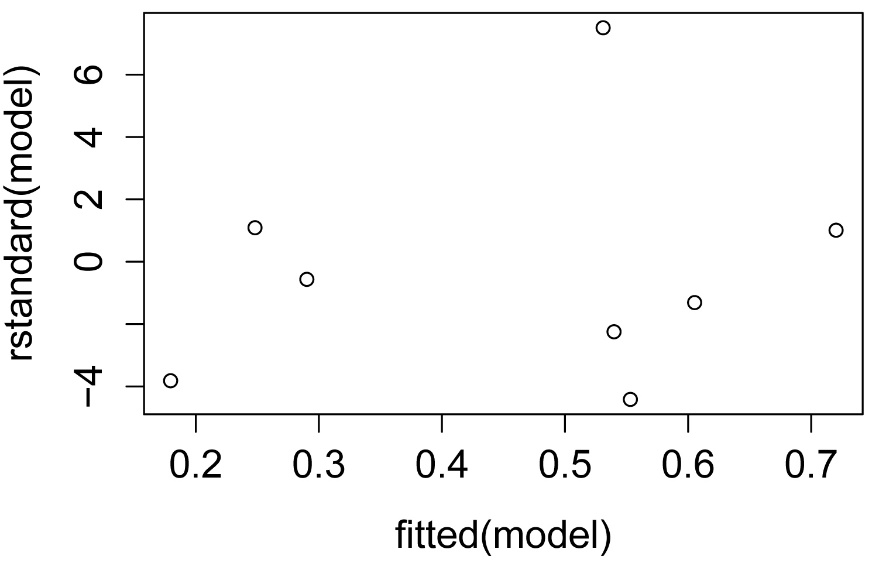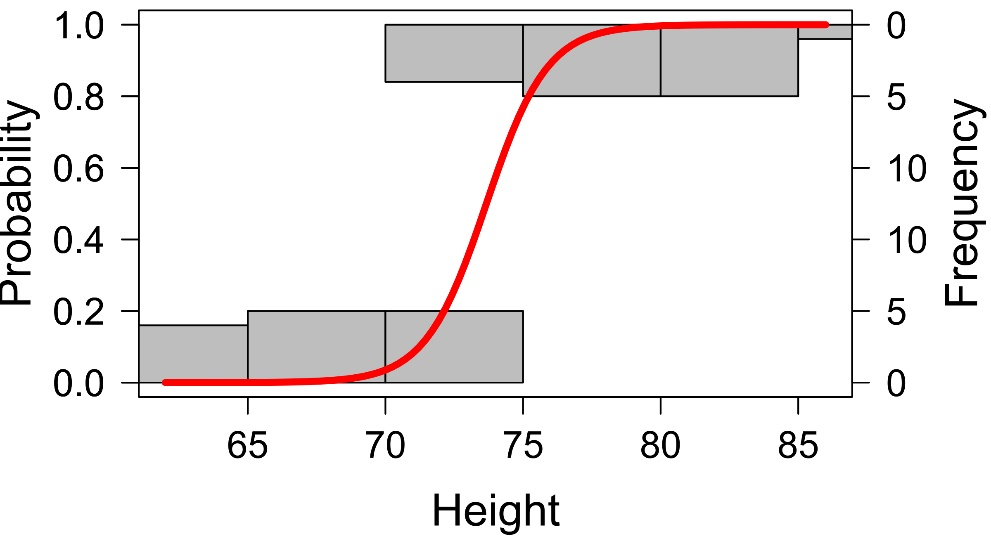## An R Companion for the Handbook of Biological Statistics

Salvatore S. Mangiafico

# Simple Logistic Regression

When to use it

Null hypothesis

How the test works

Assumptions

See the Handbook for information on these topics.

Examples

The Mpi example is shown below in the “How to do the test” section.

Graphing the results

Similar tests

See the Handbook for information on these topics.

### How to do the test

Logistic regression can be performed in R with the glm (generalized linear model) function.  This function uses a link function to determine which kind of model to use, such as logistic, probit, or poisson.  These are indicated in the family and link options.  See ?glm and ?family for more information.

##### Assumptions

Generalized linear models have fewer assumptions than most common parametric tests.  Observations still need to be independent, and the correct link function needs to be specified.  So, for example you should understand when to use a poisson regression, and when to use a logistic regression.  However, the normal distribution of data or residuals is not required.

##### Specifying the counts of “successes” and “failures”

Logistic regression has a dependent variable with two levels.  In R, this can be specified in three ways.  1) The dependent variable can be a factor variable where the first level is interpreted as “failure” and the other levels are interpreted as “success”.  (As in the second example in this chapter).  2) The dependent variable can be a vector of proportions of successes, with the caveat that the number of observations for each proportion is indicated in the weights option.  3) The dependent variable can be a matrix with two columns, with the first column being the number of “successes” and the second being the number of “failures”.  (As in the first example in this chapter).

##### Not all proportions or counts are appropriate for logistic regression analysis

Note that in each of these specifications, both the number of successes and the number of failures is known.  You should not perform logistic regression on proportion data where you don’t know (or don’t tell R) how many individuals went into those proportions.  In statistics, 75% is different if it means 3 out of 4 rather than 150 out of 200.  As another example where logistic regression doesn’t apply, the weight people lose in a diet study expressed as a proportion of initial weight cannot be interpreted as a count of “successes” and “failures”.  Here, you might be able to use common parametric methods, provided the model assumptions are met; log or arc-sine transformations may be appropriate.  Likewise, if you count the number of people in front of you in line, you can’t interpret this as a percentage of people since you don’t know how many people are not in front of you in line.  In this case with count data as the dependent variable, you might use poisson regression.

##### Overdispersion

One potential problem to be aware of when using generalized linear models is overdispersion.  This occurs when the residual deviance of the model is high relative to the residual degrees of freedom.  It is basically an indication that the model doesn’t fit the data well.

It is my understanding, however, that overdispersion is technically not a problem for a simple logistic regression, that is one with a binomial dependent and a single continuous independent variable.  Overdispersion is discussed in the chapter on Multiple logistic regression.

##### Pseudo-R-squared

R does not produce r-squared values for generalized linear models (glm).  My function nagelkerke will calculate the McFadden, Cox and Snell, and Nagelkereke pseudo-R-squared for glm and other model fits.  The Cox and Snell is also called the ML, and the Nagelkerke is also called the Cragg and Uhler.  These pseudo-R-squared values compare the maximum likelihood of the model to a nested null model fit with the same method.  They should not be thought of as the same as the r-squared from an ordinary-least-squares linear (OLS) model, but instead as a relative measure among similar models.  The Cox and Snell for an OLS linear model, however, will be equivalent to r-squared for that model.  I have seen it mentioned that a McFadden pseudo-R-squared of 0.2–0.4 indicates a good fit.  Whereas, I find that the Nagelkerke usually gives a reasonable indication of the goodness of fit for a model on a scale of 0 to 1.  That being said, I have found the Cox and Snell and Nagelkerke to sometimes yield values I wouldn’t expect for some glm.  The function pR2 in the package pscl will also produce these pseudo-R-squared values.

##### Testing for p-values

Note that testing p-values for a logistic or poisson regression uses Chi-square tests.  This is achieved through the test=“Wald” option in Anova to test the significance of each coefficient, and the test=“Chisq” option in anova for the significance of the overall model.  A likelihood ratio test can also be used to test the significance of the overall model.

#### Logistic regression example

### --------------------------------------------------------------
### Logistic regression, amphipod example, p. 247
### --------------------------------------------------------------

Input = ("
Location            Latitude  mpi90  mpi100
Port_Townsend,_WA  48.1        47    139
Neskowin,_OR       45.2       177    241
Siuslaw_R.,_OR     44.0      1087   1183
Umpqua_R.,_OR      43.7       187    175
Coos_Bay,_OR       43.5       397    671
San_Francisco,_CA  37.8        40     14
Carmel,_CA         36.6        39     17
Santa_Barbara,_CA  34.3        30      0
")

Data\$Total = Data\$mpi90 + Data\$mpi100

Data\$Percent = Data\$mpi100 / + Data\$Total

##### Model fitting

Trials = cbind(Data\$mpi100, Data\$mpi90)         # Sucesses, Failures

model = glm(Trials ~ Latitude,
data = Data,

##### Coefficients and exponentiated cofficients

summary(model)

Coefficients:

Estimate Std. Error z value Pr(>|z|)

(Intercept) -7.64686    0.92487  -8.268   <2e-16 ***

Latitude     0.17864    0.02104   8.490   <2e-16 ***

confint(model)

2.5 %     97.5 %

(Intercept) -9.5003746 -5.8702453

Latitude     0.1382141  0.2208032

exp(model\$coefficients)         # exponentiated coefficients

(Intercept)     Latitude

0.0004775391 1.1955899446

exp(confint(model))             # 95% CI for exponentiated coefficients

2.5 %      97.5 %

(Intercept) 7.482379e-05 0.002822181

Latitude    1.148221e+00 1.247077992

##### Analysis of variance for individual terms

library(car)

Anova(model, type="II", test="Wald")

Analysis of Deviance Table (Type II tests)

Response: Trials

Df  Chisq Pr(>Chisq)

Latitude   1 72.076  < 2.2e-16 ***

##### Pseudo-R-squared

library(rcompanion)

nagelkerke(model)

\$Models

Model: "glm, Trials ~ Latitude, binomial(link = \"logit\"), Data"

Null:  "glm, Trials ~ 1, binomial(link = \"logit\"), Data"

\$Pseudo.R.squared.for.model.vs.null

Pseudo.R.squared

Cox and Snell (ML)                   0.999970

Nagelkerke (Cragg and Uhler)         0.999970

##### Overall p-value for model

anova(model,
update(model, ~1),    # update here produces null model for comparison
test="Chisq")

Analysis of Deviance Table

Model 1: Trials ~ Latitude

Model 2: Trials ~ 1

Resid. Df Resid. Dev Df Deviance  Pr(>Chi)

1         6     70.333

2         7    153.633 -1  -83.301 < 2.2e-16 ***

library(lmtest)

lrtest(model)

Likelihood ratio test

Model 1: Trials ~ Latitude

Model 2: Trials ~ 1

#Df  LogLik Df  Chisq Pr(>Chisq)

1   2 -56.293

2   1 -97.944 -1 83.301  < 2.2e-16 ***

##### Plot of standardized residuals

plot(fitted(model),
rstandard(model))A plot of standardized residuals vs. predicted values.  The residuals should be unbiased and homoscedastic.  For an illustration of these properties, see this diagram by Steve Jost at DePaul University: condor.depaul.edu/sjost/it223/documents/resid-plots.gif.

### additional model checking plots with: plot(model)

##### Plotting the model

plot(Percent ~ Latitude,
data = Data,
xlab="Latitude",
ylab="Percent mpi100",
pch=19)

curve(predict(model,data.frame(Latitude=x),type="response"),
lty=1, lwd=2, col="blue",#     #     #

#### Logistic regression example

### --------------------------------------------------------------
### Logistic regression, favorite insect example, p. 248
### --------------------------------------------------------------

Input = ("
Height   Insect
62      beetle
66      other
61      beetle
67      other
62      other
76      other
66      other
70      beetle
67      other
66      other
70      other
70      other
77      beetle
76      other
72      beetle
76      beetle
72      other
70      other
65      other
63      other
63      other
70      other
72      other
70      beetle
74      other
")

##### Model fitting

model = glm(Insect ~ Height,
data=Data,

##### Coefficients and exponentiated cofficients

summary(model)

Coefficients:

Estimate Std. Error z value Pr(>|z|)

(Intercept)  4.41379    6.66190   0.663    0.508

Height      -0.05016    0.09577  -0.524    0.600

confint(model)

2.5 %     97.5 %

(Intercept) -8.4723648 18.4667731

Height      -0.2498133  0.1374819

exp(model\$coefficients)         # exponentiated coefficients

(Intercept)      Height

82.5821122   0.9510757

exp(confint(model))             # 95% CI for exponentiated coefficients

2.5 %       97.5 %

(Intercept) 0.0002091697 1.047171e+08

Height      0.7789461738 1.147381e+0

##### Analysis of variance for individual terms

library(car)

Anova(model, type="II", test="Wald")

Analysis of Deviance Table (Type II tests)

Response: Insect

Df  Chisq Pr(>Chisq)

Height     1 0.2743     0.6004

Residuals 23

##### Pseudo-R-squared

library(rcompanion)

nagelkerke(model)

\$Pseudo.R.squared.for.model.vs.null

Pseudo.R.squared

Cox and Snell (ML)                 0.01105020

Nagelkerke (Cragg and Uhler)       0.01591030

##### Overall p-value for model

anova(model,
update(model, ~1),    # update here produces null model for comparison
test="Chisq")

Analysis of Deviance Table

Model 1: Insect ~ Height

Model 2: Insect ~ 1

Resid. Df Resid. Dev Df Deviance Pr(>Chi)

1        23     29.370

2        24     29.648 -1 -0.27779   0.5982

library(lmtest)

lrtest(model)

Likelihood ratio test

Model 1: Insect ~ Height

Model 2: Insect ~ 1

#Df  LogLik Df  Chisq Pr(>Chisq)

1   2 -14.685

2   1 -14.824 -1 0.2778     0.5982

##### Plot of standardized residuals

plot(fitted(model),
rstandard(model))##### Plotting the model

###  Convert Insect to a numeric variable, levels 0 and 1

Data\$Insect.num=as.numeric(Data\$Insect)-1

library(FSA)

Height Insect Insect.num

1      62 beetle          0

2      66  other          1

3      61 beetle          0

23     72  other          1

24     70 beetle          0

25     74  other          1

### Plot

plot(Insect.num ~ Height,
data = Data,
xlab="Height",
ylab="Insect",
pch=19)

curve(predict(model,data.frame(Height=x),type="response"),
lty=1, lwd=2, col="blue",###  Convert Insect to a logical variable, levels TRUE and FALSE

Data\$Insect.log=(Data\$Insect=="other")

library(FSA)

Height Insect Insect.num Insect.log

1      62 beetle          0      FALSE

2      66  other          1       TRUE

3      61 beetle          0      FALSE

23     72  other          1       TRUE

24     70 beetle          0      FALSE

25     74  other          1       TRUE

library(popbio)

logi.hist.plot(Data\$Height,
Data\$Insect.log,
boxp=FALSE,
type="hist",
col="gray",
xlabel="Height")#     #     #

#### Logistic regression example with significant model and abbreviated code

### --------------------------------------------------------------
### Logistic regression, hypothetical example
### Abbreviated code and description
### --------------------------------------------------------------

Input = ("
Continuous  Factor
62          A
63          A
64          A
65          A
66          A
67          A
68          A
69          A
70          A
71          A
72          A
73          A
74          A
75          A
72.5        B
73.5        B
74.5        B
75          B
76          B
77          B
78          B
79          B
80          B
81          B
82          B
83          B
84          B
85          B
86          B
")

model = glm(Factor ~ Continuous,
data=Data,

summary(model)

Coefficients:

Estimate Std. Error z value Pr(>|z|)

(Intercept) -66.4981    32.3787  -2.054   0.0400 *

Continuous    0.9027     0.4389   2.056   0.0397 *

library(car)

Anova(model, type="II", test="Wald")

Analysis of Deviance Table (Type II tests)

Response: Factor

Df Chisq Pr(>Chisq)

Continuous  1 4.229    0.03974 *

Residuals  27

library(rcompanion)

nagelkerke(model)

Pseudo.R.squared

Cox and Snell (ML)                   0.619482

Nagelkerke (Cragg and Uhler)         0.826303

anova(model,
update(model, ~1),
test="Chisq")

Resid. Df Resid. Dev Df Deviance Pr(>Chi)

1        27     12.148

2        28     40.168 -1   -28.02  1.2e-07 ***

plot(fitted(model),
rstandard(model))###  Convert Factor to a numeric variable, levels 0 and 1

Data\$Factor.num=as.numeric(Data\$Factor)-1

library(FSA)

Continuous Factor Factor.num

1          62      A          0

2          63      A          0

3          64      A          0

27         84      B          1

28         85      B          1

29         86      B          1

plot(Factor.num  ~ Continuous,
data = Data,
xlab="Continuous",
ylab="Factor",
pch=19)

curve(predict(model,data.frame(Continuous=x),type="response"),
lty=1, lwd=2, col="blue",###  Convert Factor to a logical variable, levels TRUE and FALSE

Data\$Factor.log=(Data\$Factor=="B")

library(FSA)

Continuous Factor Factor.num Factor.log

1          62      A          0      FALSE

2          63      A          0      FALSE

3          64      A          0      FALSE

27         84      B          1       TRUE

28         85      B          1       TRUE

29         86      B          1       TRUE

library(popbio)

logi.hist.plot(Data\$Continuous,
Data\$Factor.log,
boxp=FALSE,
type="hist",
col="gray",
xlabel="Height")#     #     #

Power analysis

See the Handbook for information on this topic.